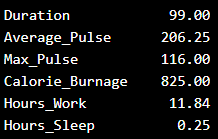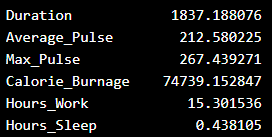# Data Science - Statistics Variance

## Variance

Variance is another number that indicates how spread out the values are.

In fact, if you take the square root of the variance, you get the standard deviation. Or the other way around, if you multiply the standard deviation by itself, you get the variance!

We will first use the data set with 10 observations to give an example of how we can calculate the variance:

Duration Average_Pulse Max_Pulse Calorie_Burnage Hours_Work Hours_Sleep
30 80 120 240 10 7
30 85 120 250 10 7
45 90 130 260 8 7
45 95 130 270 8 7
45 100 140 280 0 7
60 105 140 290 7 8
60 110 145 300 7 8
60 115 145 310 8 8
75 120 150 320 0 8
75 125 150 330 8 8

Tip: Variance is often represented by the symbol Sigma Square: σ^2

## Step 1 to Calculate the Variance: Find the Mean

We want to find the variance of Average_Pulse.

1. Find the mean:

(80+85+90+95+100+105+110+115+120+125) / 10 = 102.5

The mean is 102.5

## Step 2: For Each Value - Find the Difference From the Mean

2. Find the difference from the mean for each value:

80 - 102.5 = -22.5
85 - 102.5 = -17.5
90 - 102.5 = -12.5
95 - 102.5 = -7.5
100 - 102.5 = -2.5
105 - 102.5 = 2.5
110 - 102.5 = 7.5
115 - 102.5 = 12.5
120 - 102.5 = 17.5
125 - 102.5 = 22.5

## Step 3: For Each Difference - Find the Square Value

3. Find the square value for each difference:

(-22.5)^2 = 506.25
(-17.5)^2 = 306.25
(-12.5)^2 = 156.25
(-7.5)^2 = 56.25
(-2.5)^2 = 6.25
2.5^2 = 6.25
7.5^2 = 56.25
12.5^2 = 156.25
17.5^2 = 306.25
22.5^2 = 506.25

Note: We must square the values to get the total spread.

## Step 4: The Variance is the Average Number of These Squared Values

4. Sum the squared values and find the average:

(506.25 + 306.25 + 156.25 + 56.25 + 6.25 + 6.25 + 56.25 + 156.25 + 306.25 + 506.25) / 10 = 206.25

The variance is 206.25.

## Use Python to Find the Variance of health_data

We can use the `var()` function from Numpy to find the variance (remember that we now use the first data set with 10 observations):

### Example

import numpy as np

var = np.var(health_data)
print(var)
Try it Yourself »

The output:## Use Python to Find the Variance of Full Data Set

Here we calculate the variance for each column for the full data set:

### Example

import numpy as np

var_full = np.var(full_health_data)
print(var_full)
Try it Yourself »

The output:W3Schools is optimized for learning and training. Examples might be simplified to improve reading and learning. Tutorials, references, and examples are constantly reviewed to avoid errors, but we cannot warrant full correctness of all content. While using W3Schools, you agree to have read and accepted our terms of use, cookie and privacy policy.